# FirstOrder Logic Review 1 RDFSOWL Semantics The semantics

• Slides: 27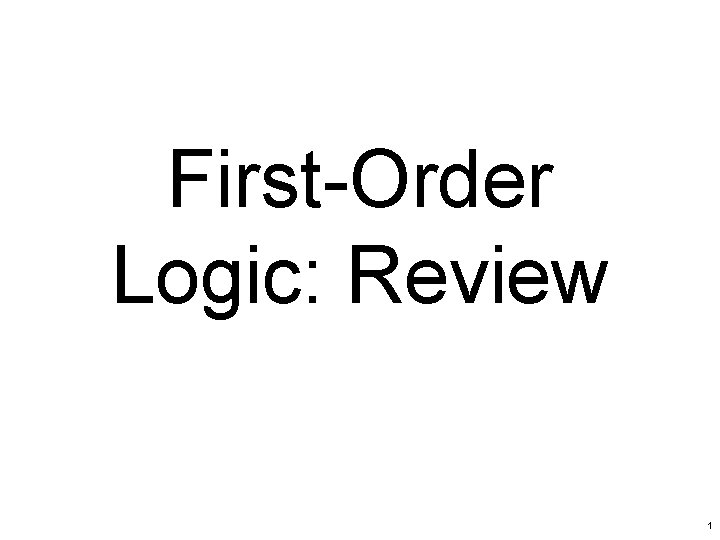First-Order Logic: Review 1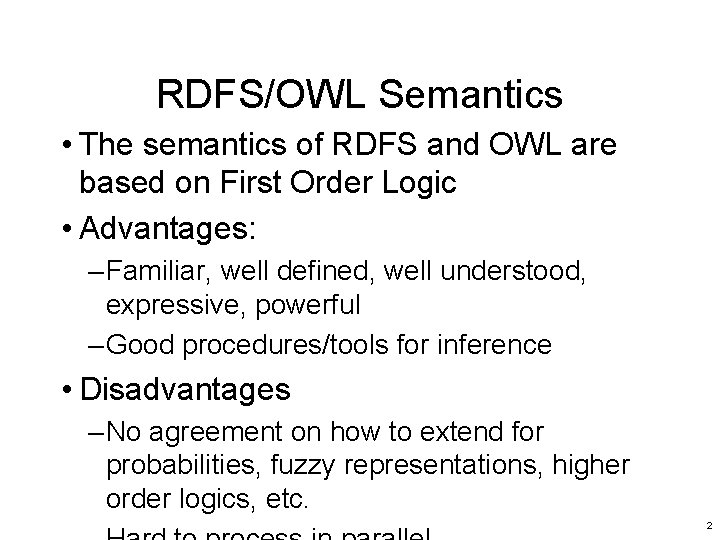RDFS/OWL Semantics • The semantics of RDFS and OWL are based on First Order Logic • Advantages: – Familiar, well defined, well understood, expressive, powerful – Good procedures/tools for inference • Disadvantages – No agreement on how to extend for probabilities, fuzzy representations, higher order logics, etc. 2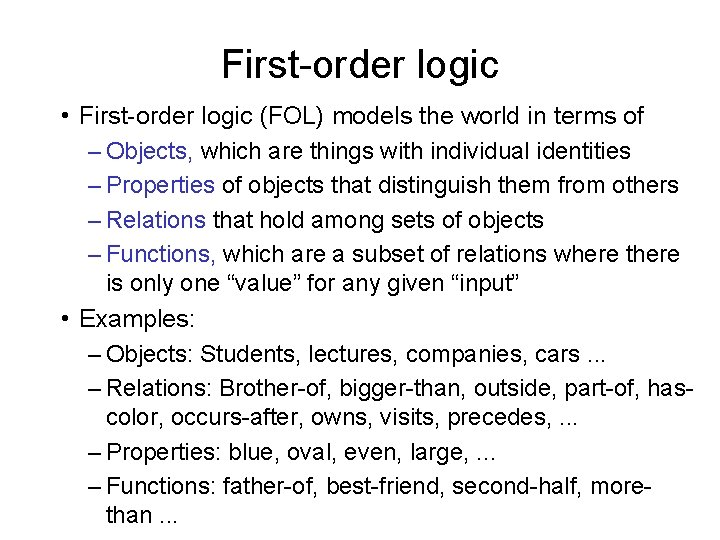First-order logic • First-order logic (FOL) models the world in terms of – Objects, which are things with individual identities – Properties of objects that distinguish them from others – Relations that hold among sets of objects – Functions, which are a subset of relations where there is only one “value” for any given “input” • Examples: – Objects: Students, lectures, companies, cars. . . – Relations: Brother-of, bigger-than, outside, part-of, hascolor, occurs-after, owns, visits, precedes, . . . – Properties: blue, oval, even, large, . . . – Functions: father-of, best-friend, second-half, morethan. . .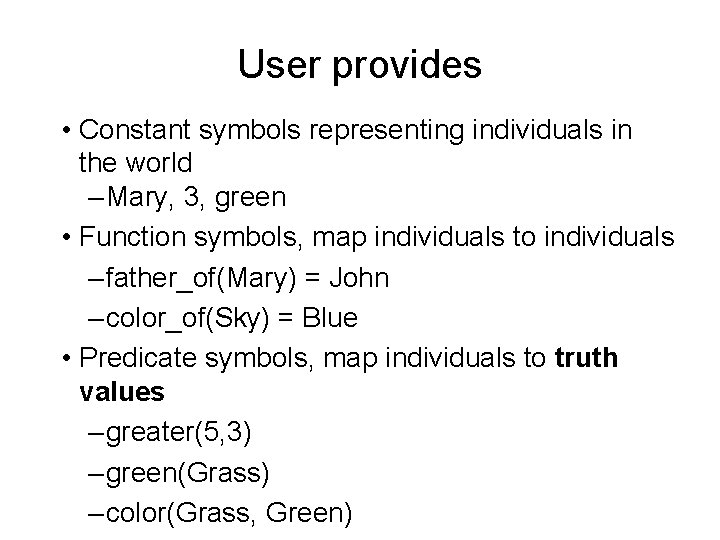User provides • Constant symbols representing individuals in the world – Mary, 3, green • Function symbols, map individuals to individuals – father_of(Mary) = John – color_of(Sky) = Blue • Predicate symbols, map individuals to truth values – greater(5, 3) – green(Grass) – color(Grass, Green)FOL Provides • Truth values – True, False • Variable symbols – E. g. , x, y, foo • Connectives – Same as in propositional logic: not ( ), and ( ), or ( ), implies ( ), iff ( ) • Quantifiers – Universal x or (Ax) – Existential x or (Ex)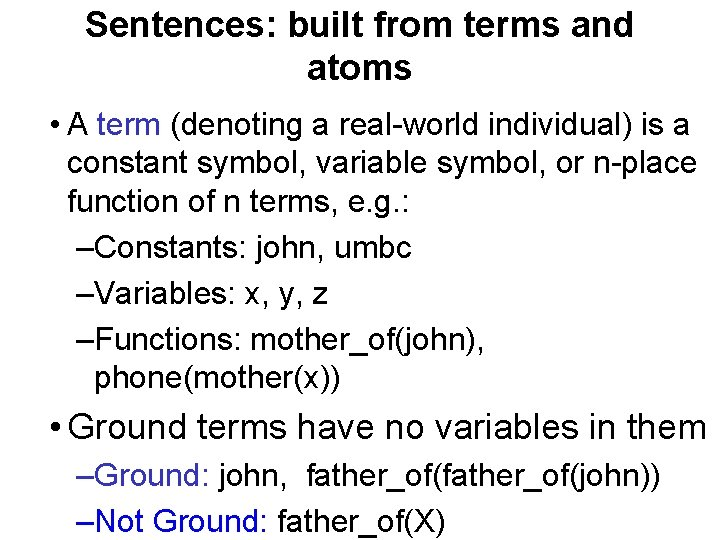Sentences: built from terms and atoms • A term (denoting a real-world individual) is a constant symbol, variable symbol, or n-place function of n terms, e. g. : –Constants: john, umbc –Variables: x, y, z –Functions: mother_of(john), phone(mother(x)) • Ground terms have no variables in them –Ground: john, father_of(john)) –Not Ground: father_of(X)Sentences: built from terms and atoms • An atomic sentence (which has value true or false) is an n-place predicate of n terms, e. g. : –green(Kermit)) –between(Philadelphia, Baltimore, DC) –loves(X, mother(X)) • A complex sentence is formed from atomic sentences connected by logical connectives: P, P Q, P Q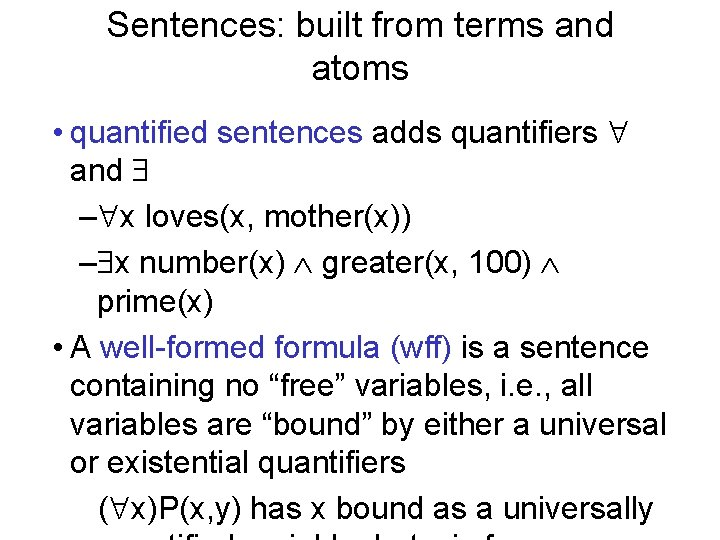Sentences: built from terms and atoms • quantified sentences adds quantifiers and – x loves(x, mother(x)) – x number(x) greater(x, 100) prime(x) • A well-formed formula (wff) is a sentence containing no “free” variables, i. e. , all variables are “bound” by either a universal or existential quantifiers ( x)P(x, y) has x bound as a universallyQuantifiers • Universal quantification –( x)P(x) means P holds for all values of x in domain associated with variable –E. g. , ( x) dolphin(x) mammal(x) • Existential quantification –( x)P(x) means P holds for some value of x in domain associated with variable –E. g. , ( x) mammal(x) lays_eggs(x) –This lets us make a statement about some object without naming itQuantifiers (1) • Universal quantifiers often used with implies to form rules: ( x) student(x) smart(x) means “All students are smart” • Universal quantification rarely used to make blanket statements about every individual in the world: ( x) student(x) smart(x) means “Everyone in the world is a student and is smart”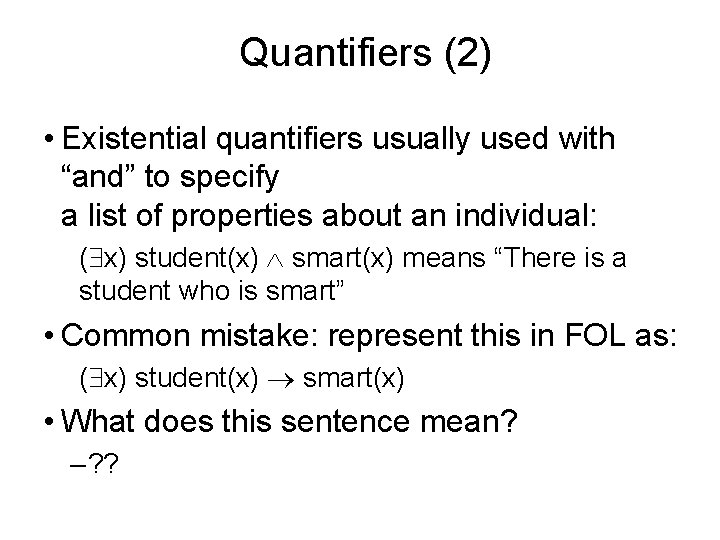Quantifiers (2) • Existential quantifiers usually used with “and” to specify a list of properties about an individual: ( x) student(x) smart(x) means “There is a student who is smart” • Common mistake: represent this in FOL as: ( x) student(x) smart(x) • What does this sentence mean? – ? ?Quantifier Scope • FOL sentences have structure, like programs • In particular, the variables in a sentence have a scope • For example, suppose we want to say – “everyone who is alive loves someone” – ( x) alive(x) ( y) loves(x, y) • Here’s how we scope the variables ( x) alive(x) ( y) loves(x, y) Scope of x Scope of y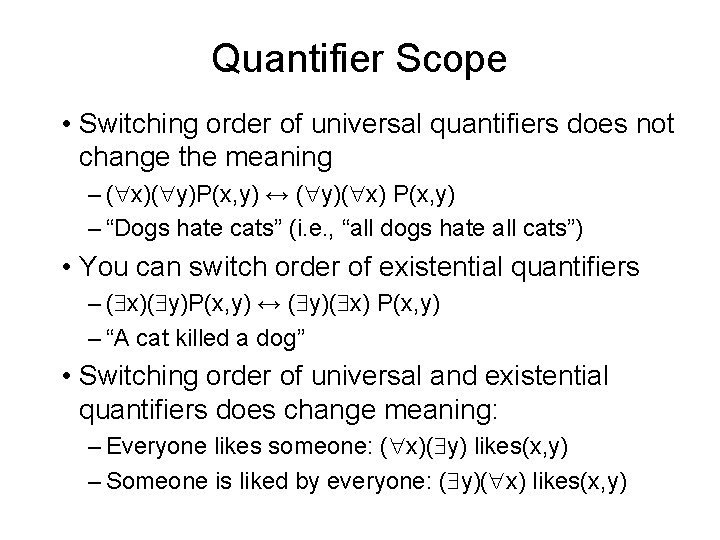Quantifier Scope • Switching order of universal quantifiers does not change the meaning – ( x)( y)P(x, y) ↔ ( y)( x) P(x, y) – “Dogs hate cats” (i. e. , “all dogs hate all cats”) • You can switch order of existential quantifiers – ( x)( y)P(x, y) ↔ ( y)( x) P(x, y) – “A cat killed a dog” • Switching order of universal and existential quantifiers does change meaning: – Everyone likes someone: ( x)( y) likes(x, y) – Someone is liked by everyone: ( y)( x) likes(x, y)Procedural example 1 def verify 1(): # Everyone likes someone: ( x)( y) likes(x, y) for x in people(): found = False for y in people(): if likes(x, y): Every person has at least one individual that found = True they like. break if not Found: return False return TrueProcedural example 2 def verify 2(): # Someone is liked by everyone: ( y)( x) likes(x, y) for y in people(): found = True for x in people(): There is a person who is if not likes(x, y): liked by every person in the universe. found = False break if found return True return FalseConnections between and • We can relate sentences involving and using extensions to De Morgan’s laws: 1. ( x) P(x) ↔ ( x) P(x) 2. ( x) P(x) ↔ ( x) P(x) 3. ( x) P(x) ↔ ( x) P(x) 4. ( x) P(x) ↔ ( x) P(x) • Examples 1. All dogs don’t like cats ↔ No dogs like cats 2. Not all dogs dance ↔ There is a dog that doesn’t dance 3. All dogs sleep ↔ There is no dog that doesn’t sleep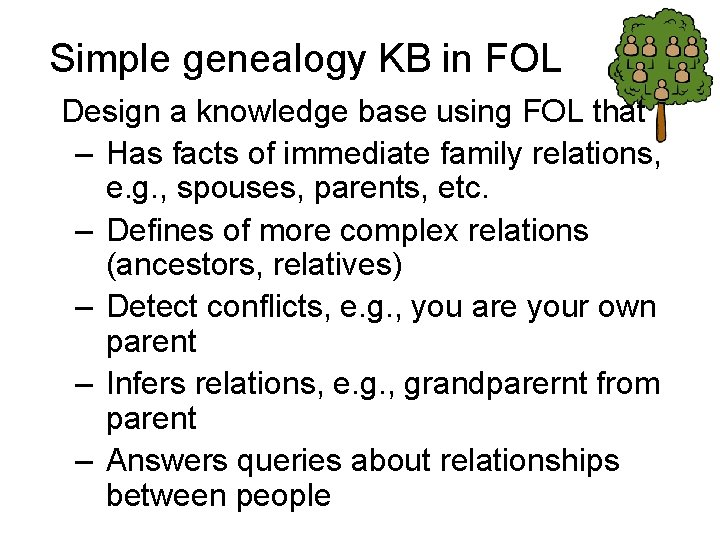Simple genealogy KB in FOL Design a knowledge base using FOL that – Has facts of immediate family relations, e. g. , spouses, parents, etc. – Defines of more complex relations (ancestors, relatives) – Detect conflicts, e. g. , you are your own parent – Infers relations, e. g. , grandparernt from parent – Answers queries about relationships between people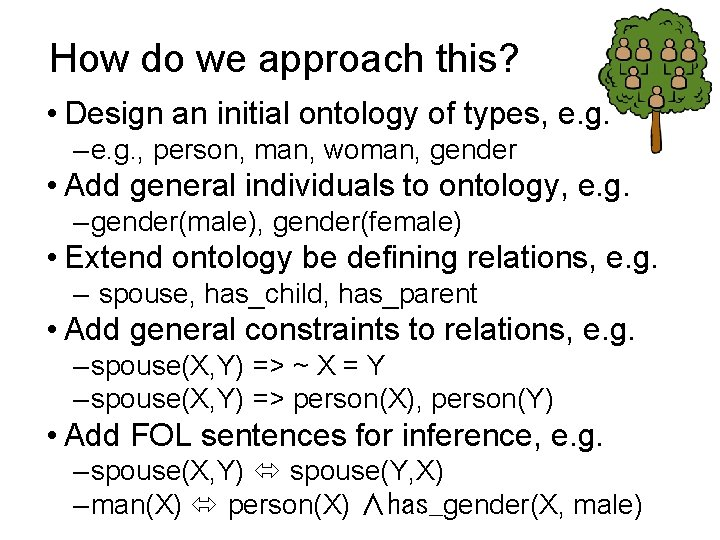How do we approach this? • Design an initial ontology of types, e. g. – e. g. , person, man, woman, gender • Add general individuals to ontology, e. g. – gender(male), gender(female) • Extend ontology be defining relations, e. g. – spouse, has_child, has_parent • Add general constraints to relations, e. g. – spouse(X, Y) => ~ X = Y – spouse(X, Y) => person(X), person(Y) • Add FOL sentences for inference, e. g. – spouse(X, Y) spouse(Y, X) – man(X) person(X) ∧has_gender(X, male)Simple genealogy KB in FOL • Has facts of immediate family relations, e. g. , spouses, parents, etc. • Has definitions of more complex relations (ancestors, relatives) • Can detect conflicts, e. g. , you are your own parent • Can infer relations, e. g. , grandparernt from parent • Can answer queries about relationships between peopleExample: A simple genealogy KB by FOL • Predicates: – parent(x, y), child(x, y), father(x, y), daughter(x, y), etc. – spouse(x, y), husband(x, y), wife(x, y) – ancestor(x, y), descendant(x, y) – male(x), female(y) – relative(x, y) • Facts: – husband(Joe, Mary), son(Fred, Joe) – spouse(John, Nancy), male(John), son(Mark, Nancy) – father(Jack, Nancy), daughter(Linda, Jack) – daughter(Liz, Linda) – etc.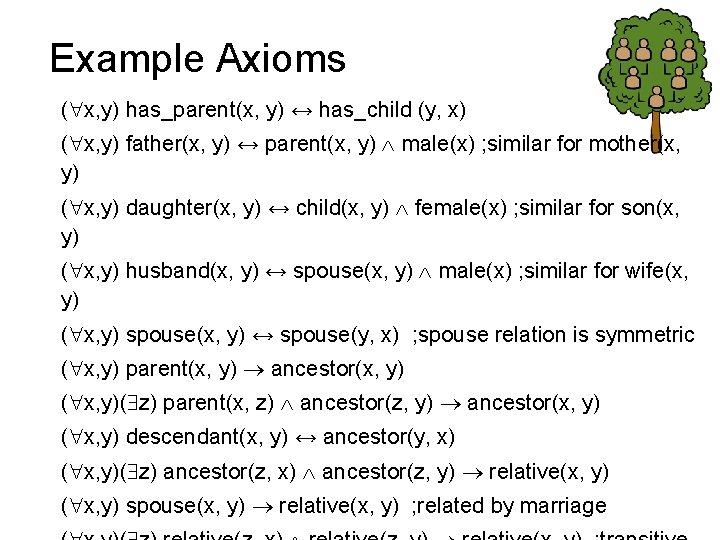Example Axioms ( x, y) has_parent(x, y) ↔ has_child (y, x) ( x, y) father(x, y) ↔ parent(x, y) male(x) ; similar for mother(x, y) ( x, y) daughter(x, y) ↔ child(x, y) female(x) ; similar for son(x, y) ( x, y) husband(x, y) ↔ spouse(x, y) male(x) ; similar for wife(x, y) ( x, y) spouse(x, y) ↔ spouse(y, x) ; spouse relation is symmetric ( x, y) parent(x, y) ancestor(x, y) ( x, y)( z) parent(x, z) ancestor(z, y) ancestor(x, y) ( x, y) descendant(x, y) ↔ ancestor(y, x) ( x, y)( z) ancestor(z, x) ancestor(z, y) relative(x, y) ( x, y) spouse(x, y) relative(x, y) ; related by marriage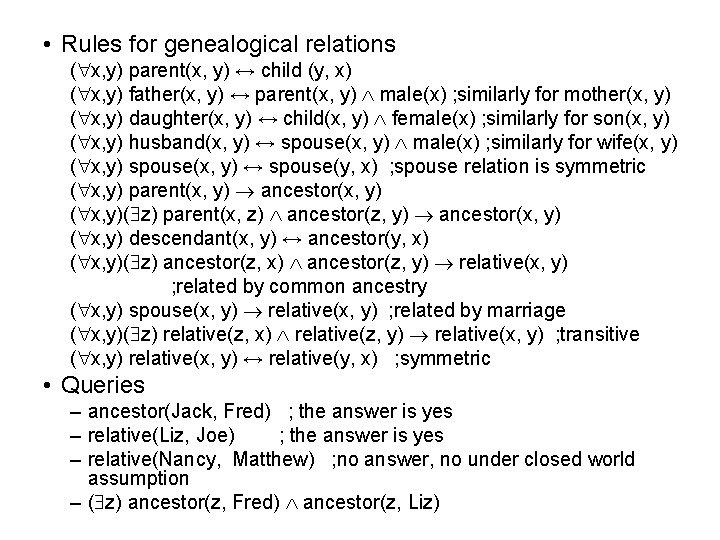• Rules for genealogical relations ( x, y) parent(x, y) ↔ child (y, x) ( x, y) father(x, y) ↔ parent(x, y) male(x) ; similarly for mother(x, y) ( x, y) daughter(x, y) ↔ child(x, y) female(x) ; similarly for son(x, y) ( x, y) husband(x, y) ↔ spouse(x, y) male(x) ; similarly for wife(x, y) ( x, y) spouse(x, y) ↔ spouse(y, x) ; spouse relation is symmetric ( x, y) parent(x, y) ancestor(x, y) ( x, y)( z) parent(x, z) ancestor(z, y) ancestor(x, y) ( x, y) descendant(x, y) ↔ ancestor(y, x) ( x, y)( z) ancestor(z, x) ancestor(z, y) relative(x, y) ; related by common ancestry ( x, y) spouse(x, y) relative(x, y) ; related by marriage ( x, y)( z) relative(z, x) relative(z, y) relative(x, y) ; transitive ( x, y) relative(x, y) ↔ relative(y, x) ; symmetric • Queries – ancestor(Jack, Fred) ; the answer is yes – relative(Liz, Joe) ; the answer is yes – relative(Nancy, Matthew) ; no answer, no under closed world assumption – ( z) ancestor(z, Fred) ancestor(z, Liz)Axioms, definitions and theorems • Axioms: facts and rules that capture the (important) facts and concepts about a domain; axioms can be used to prove theorems – Mathematicians dislike unnecessary (dependent) axioms, i. e. ones that can be derived from others – Dependent axioms can make reasoning faster, however – Choosing a good set of axioms is a design problem • A definition of a predicate is of the form “p(X) ↔ …” and can be decomposed into two parts – Necessary description: “p(x) …” – Sufficient description “p(x) …” – Some concepts have definitions (triangle) and some do not (person)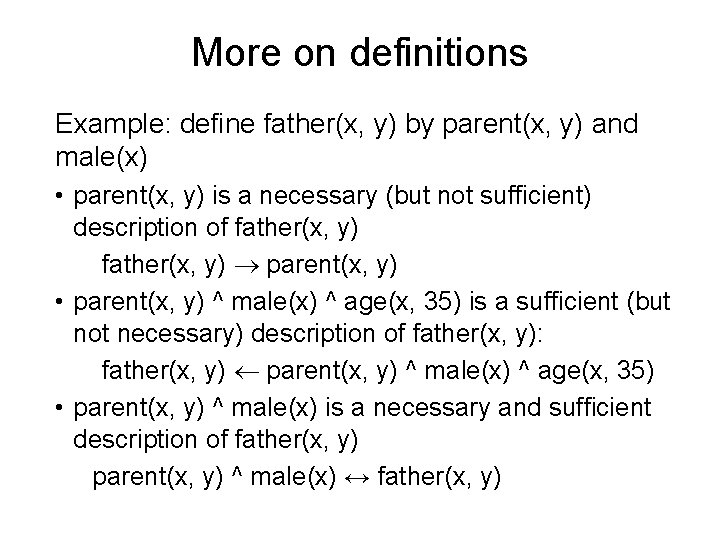More on definitions Example: define father(x, y) by parent(x, y) and male(x) • parent(x, y) is a necessary (but not sufficient) description of father(x, y) parent(x, y) • parent(x, y) ^ male(x) ^ age(x, 35) is a sufficient (but not necessary) description of father(x, y): father(x, y) parent(x, y) ^ male(x) ^ age(x, 35) • parent(x, y) ^ male(x) is a necessary and sufficient description of father(x, y) parent(x, y) ^ male(x) ↔ father(x, y)Notational differences • Different symbols for and, or, not, implies, . . . – – p v (q ^ r) – p + (q * r) • Prolog cat(X) : - furry(X), meows (X), has(X, claws) • Lispy notations (forall ? x (implies (and (furry ? x) (meows ? x) (has ? x claws))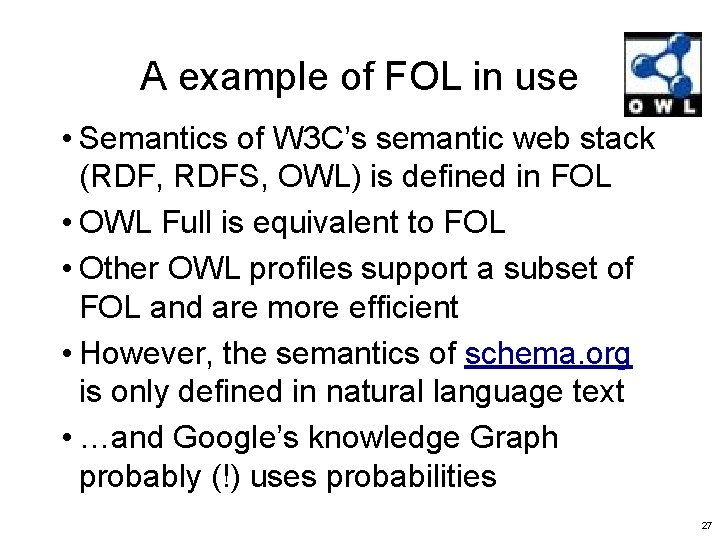A example of FOL in use • Semantics of W 3 C’s semantic web stack (RDF, RDFS, OWL) is defined in FOL • OWL Full is equivalent to FOL • Other OWL profiles support a subset of FOL and are more efficient • However, the semantics of schema. org is only defined in natural language text • …and Google’s knowledge Graph probably (!) uses probabilities 27FOL Summary • First order logic (FOL) introduces predicates, functions and quantifiers • More expressive, but reasoning more complex – Reasoning in propositional logic is NP hard, FOL is semi-decidable • Common AI knowledge representation language – Other KR languages (e. g. , OWL) are often defined by mapping them to FOL • FOL variables range over objects